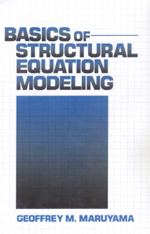# Basics of Structural Equation Modeling

With the availability of software programs such as LISREL, EQS, and AMOS modeling techniques have become a popular tool for formalized presentation of the hypothesized relationships underlying correlational research and for testing the plausibility of hypothesizing for a particular data set. The popularity of these techniques, however, has often led to misunderstandings of them, particularly by students being exposed to them for the first time. Through the use of careful narrative explanation, Basics of Structural Equation Modeling describes the logic underlying structural equation modeling (SEM) approaches, describes how SEM approaches relate to techniques like regression and factor analysis, analyzes the strengths and shortcomings of SEM as compared to alternative methodologies, and explores the various methodologies for analyzing structural equation data.

•••